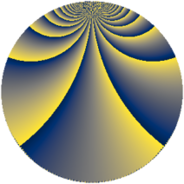# Properties

 Label 384.5.xLevel $384$ Weight $5$ Character orbit 384.x Rep. character $\chi_{384}(5,\cdot)$ Character field $\Q(\zeta_{32})$ Dimension $4064$ Sturm bound $320$

# Related objects

## Defining parameters

 Level: $$N$$ $$=$$ $$384 = 2^{7} \cdot 3$$ Weight: $$k$$ $$=$$ $$5$$ Character orbit: $$[\chi]$$ $$=$$ 384.x (of order $$32$$ and degree $$16$$) Character conductor: $$\operatorname{cond}(\chi)$$ $$=$$ $$384$$ Character field: $$\Q(\zeta_{32})$$ Sturm bound: $$320$$

## Dimensions

The following table gives the dimensions of various subspaces of $$M_{5}(384, [\chi])$$.

Total New Old
Modular forms 4128 4128 0
Cusp forms 4064 4064 0
Eisenstein series 64 64 0

## Trace form

 $$4064 q - 16 q^{3} - 32 q^{4} - 16 q^{6} - 32 q^{7} - 16 q^{9} + O(q^{10})$$ $$4064 q - 16 q^{3} - 32 q^{4} - 16 q^{6} - 32 q^{7} - 16 q^{9} - 32 q^{10} - 16 q^{12} - 32 q^{13} - 16 q^{15} - 32 q^{16} - 16 q^{18} - 32 q^{19} - 16 q^{21} - 32 q^{22} - 16 q^{24} - 32 q^{25} - 16 q^{27} - 32 q^{28} - 16 q^{30} - 32 q^{31} - 16 q^{33} - 32 q^{34} - 16 q^{36} - 32 q^{37} - 16 q^{39} - 32 q^{40} - 16 q^{42} - 32 q^{43} - 16 q^{45} - 32 q^{46} - 16 q^{48} - 32 q^{49} - 16 q^{51} + 17920 q^{52} - 16 q^{54} - 32 q^{55} - 16 q^{57} - 131072 q^{58} - 16 q^{60} - 32 q^{61} + 24352 q^{64} - 16 q^{66} - 32 q^{67} - 16 q^{69} + 122272 q^{70} - 16 q^{72} - 32 q^{73} - 16 q^{75} - 49536 q^{76} - 16 q^{78} - 32 q^{79} - 16 q^{81} - 32 q^{82} - 16 q^{84} - 32 q^{85} - 16 q^{87} - 32 q^{88} - 16 q^{90} - 32 q^{91} - 16 q^{93} - 32 q^{94} - 16 q^{96} - 32 q^{97} - 16 q^{99} + O(q^{100})$$

## Decomposition of $$S_{5}^{\mathrm{new}}(384, [\chi])$$ into newform subspaces

The newforms in this space have not yet been added to the LMFDB.